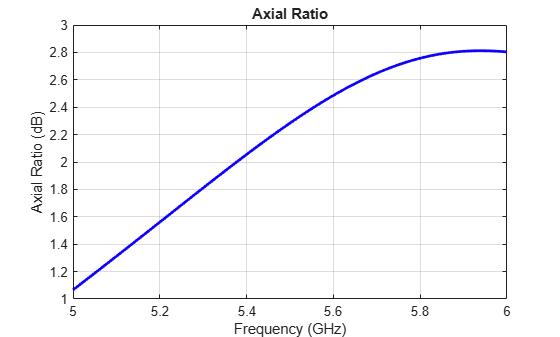# axialRatio

Axial ratio of antenna

## Syntax

``axialRatio(antenna,frequency,azimuth,elevation)``
``ar = axialRatio(antenna,frequency,azimuth,elevation)``

## Description

example

````axialRatio(antenna,frequency,azimuth,elevation)` plots axial ratio of an antenna over a specified frequency, and in the direction specified by `azimuth` and `elevation`. Any one among frequency, azimuth, or elevation values must be scalar. If only one of the values are scalar, the plot is 3-D. If two values are scalar, the plot is 2-D.```

example

````ar = axialRatio(antenna,frequency,azimuth,elevation)` returns the axial ratio of an antenna, over the specified frequency, and in the direction specified by `azimuth` and `elevation`.```

## Examples

collapse all

Calculate the axial ratio of an equiangular spiral antenna at azimuth=0 and elevation=0.

```s = spiralEquiangular; ar = axialRatio(s,3e9,0,0)```
```ar = Inf ```

Create a cloverleaf antenna.

```cl = cloverleaf; show(cl);```Plot the axial ratio of the antenna from 5 GHz to 6 GHz.

```freq = linspace(5e9,6e9,101); axialRatio(cl,freq,0,0);```The axial ratio plot shows that the antenna supports circular polarization over the entire frequency range.

## Input Arguments

collapse all

Antenna object, specified as an object.

Frequency used to calculate axial ratio, specified as a scalar or vector with each element in Hz.

Example: 70e6

Data Types: `double`

Azimuth angle of antenna, specified as a scalar or vector with each element in degrees.

Example: 0

Data Types: `double`

Elevation angle of antenna, specified as a scalar or vector with each element in degrees.

Example: 0

Data Types: `double`

## Output Arguments

collapse all

Axial ratio of antenna, returned as a scalar in dB.